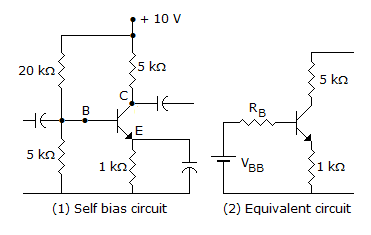# Electronics and Communication Engineering - Analog Electronics

11.

Assertion (A): An op-amp has high voltage gain, high input impedance and low output impedance

Reason (R): Negative feedback increases output impedance

 A. Both A and R are correct and R is correct explanation for A B. Both A and R are correct but R is not correct explanation for A C. A is correct R is wrong D. A is wrong R is correct

Explanation:

Negative feedback reduces output impedance.

12.

A transistor has a maximum power dissipation of 350 mW at an ambient temperature of 25°C. If derating factor is 2 mW/°C, the maximum power dissipation for 40°C ambient temperature is

 A. 300 mW B. 330 mW C. 350 mW D. 380 mW

Explanation:

Derating improves reliability but does not affect maximum power dissipation.

13.

The output voltage waveform of a CE amplifier is fed to a dc coupled CRO. The trace on the screen will be

 A. dc output voltage B. ac output voltage C. sum of dc and ac output voltage D. either (a) or (b)

Explanation:

Due to direct coupling between output and CRO both dc and ac output voltage will appear on screen of CRO.

14.

In an RC phase shift oscillator, the total phase shift of the three RC lead networks is

 A. 360° B. 180° C. 90° D. 0°

Explanation:

Each RC network gives 60° phase shift.

15.

In the circuit of figure β = 100 and quiescent value of base current is 20 μA. The quiescent value of collectorcurrent is

 A. 0.2 μA B. 2 μA C. 2 mA D. 20 mA